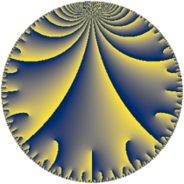Properties

 Label 126.9.oLevel $126$ Weight $9$ Character orbit 126.o Rep. character $\chi_{126}(13,\cdot)$ Character field $\Q(\zeta_{6})$ Dimension $128$ Sturm bound $216$

Related objects

Defining parameters

 Level: $$N$$ $$=$$ $$126 = 2 \cdot 3^{2} \cdot 7$$ Weight: $$k$$ $$=$$ $$9$$ Character orbit: $$[\chi]$$ $$=$$ 126.o (of order $$6$$ and degree $$2$$) Character conductor: $$\operatorname{cond}(\chi)$$ $$=$$ $$63$$ Character field: $$\Q(\zeta_{6})$$ Sturm bound: $$216$$

Dimensions

The following table gives the dimensions of various subspaces of $$M_{9}(126, [\chi])$$.

Total New Old
Modular forms 392 128 264
Cusp forms 376 128 248
Eisenstein series 16 0 16

Trace form

 $$128 q - 8192 q^{4} + 1846 q^{7} - 12216 q^{9} + O(q^{10})$$ $$128 q - 8192 q^{4} + 1846 q^{7} - 12216 q^{9} - 11388 q^{11} + 31488 q^{14} - 187584 q^{15} - 1048576 q^{16} - 271872 q^{18} - 11394 q^{21} - 644172 q^{23} + 5000000 q^{25} - 472576 q^{28} + 2541912 q^{29} + 1122816 q^{30} - 7017852 q^{35} - 188928 q^{36} + 2187944 q^{37} - 3438036 q^{39} - 10660608 q^{42} + 2262656 q^{43} + 2915328 q^{44} - 14834688 q^{46} - 3341302 q^{49} + 12025344 q^{50} + 20020308 q^{51} - 77071392 q^{53} + 4030464 q^{56} - 33120420 q^{57} - 15705600 q^{58} + 46290432 q^{60} - 61218474 q^{63} + 268435456 q^{64} - 58777008 q^{65} + 44299780 q^{67} - 34359552 q^{70} + 37894440 q^{71} - 4325376 q^{72} + 6299136 q^{74} - 70986138 q^{77} - 37324800 q^{78} - 128064440 q^{79} - 43707744 q^{81} + 48964608 q^{84} + 57202500 q^{85} + 206112768 q^{86} + 188753412 q^{91} - 82454016 q^{92} + 683945832 q^{93} + 622484304 q^{95} - 86599680 q^{98} - 84953916 q^{99} + O(q^{100})$$

Decomposition of $$S_{9}^{\mathrm{new}}(126, [\chi])$$ into newform subspaces

The newforms in this space have not yet been added to the LMFDB.

Decomposition of $$S_{9}^{\mathrm{old}}(126, [\chi])$$ into lower level spaces

$$S_{9}^{\mathrm{old}}(126, [\chi]) \cong$$ $$S_{9}^{\mathrm{new}}(63, [\chi])$$$$^{\oplus 2}$$